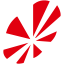#FreeStyler Wiki

### Site Tools

fixture_creator:fixture_formula

# Fixture Creator - The "Formula" Feature

The “Formula” feature can both greatly simplify a fixture's Macro window and add more controls at the same time.

### Variables

Three main variable families are available:

• GIx (GI1, GI2, GI3…. or GI01, GI02, GI03…)Gobo Index number
• CIx (CI1, CI2…. or CI01, CI02…)Color Index number
• CHx (CH1, CH2, CH3…. or CH01, CH02, CH03….) → The fixture's Channel number

NOTES:

1. GIx and CIx are indexed values and only go in the order 1, 2, 3, 4…. (sequential whole numbers)
2. GIx and CIx correspond with the number of Gobos and Colors you have set on the “Fixture Config” tab. If you have specified that Gobo Channel 1 has eight gobos, then GI01 (or GI1) will vary between 1~8 according to whichever Gobo is currently displayed. Likewise, if you have specified your Colorwheel has 12 colors, then CI01 (or CI1) will vary between 1~12.
3. CHx corresponds to the channels you have setup and described on the “Fixture Config” and “Channel Description” tabs.

### Operators

Basic math operators can be used in Formulas:

• + → Addition
• - → Subtraction
• * → Multiply
• / → Divide
• ( ) → Parenthetical Ordering

### Usage

Formulas are written in standard programming syntax. Computations on the right side of the “=” are assigned to the variable on the left side.

NOTES:

1. Spaces are NOT permitted in Formulas!
• CH01 = 6 + (GI1 * 6) ← WRONG, will not work.
• CH01=6+(GI1*6) ← CORRECT, will work. (No spaces in Formula.)
2. Only ONE “=” is permitted in a Formula. Using multiple “=” in a Formula will yield unexpected results.
• CH01=48 ← CORRECT, will work. (Assigns the value “48” to Channel 1.)
• CH01=CH02=CH03=48 ← WRONG, will not work. The programmer is trying to assign the value “48” to Channels 1, 2 and 3 simultaneously, but this will not work.

Example 1:

CH01=48

Action: This assigns the value “48” to the fixture's Channel 1.

Example 2:

CH01=GI01*16

Action: This takes the index value of Gobo Channel 1, multiplies it by 16, and assigns it to the fixture's Channel 1.

If the fixture has 4 gobos, then GI01 will range in values 1, 2, 3, or 4; and the results of this formula will assign the values 16, 32, 48, or 64 to Channel 1.

Example 3:

CH01=16*(GI01-1)

Action: This subtracts 1 from the index value of Gobo Channel 1, multiplies the result by 16, and assigns it to the fixture's Channel 1.

If the fixture has 4 gobos, then GI01 will range in values 1, 2, 3, or 4; and the results of this formula will assign the values 0, 16, 32, or 48 to Channel 1.

Example 4:

CH01=16*(GI01-1)+4

Action: This subtracts 1 from the index value of Gobo Channel 1, multiplies the result by 16, adds 4, and assigns it to the fixture's Channel 1.

If the fixture has 4 gobos, then GI01 will range in values 1, 2, 3, or 4; and the results of this formula will assign the values 4, 20, 38, or 52 to Channel 1.

Example 5:

CH2=CH1

Action: This assigns the value in Channel 1 to the fixture's Channel 2. (Programming syntax reads from right to left.)

# Summary

The new “Formula” feature in Fixture Creator and FreeStyler helps to both simplify macros and add more control. Formulas must be written following strict syntax rules, otherwise they do not work.

fixture_creator/fixture_formula.txt · Last modified: 2013/03/11 03:45 (external edit)

### Page Tools